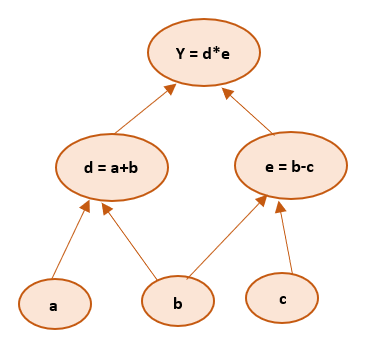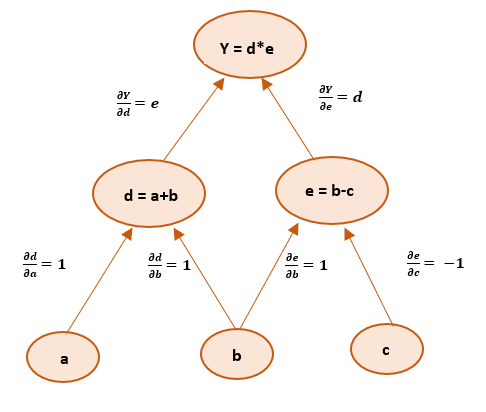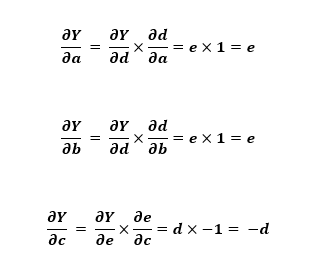# Computational Graphs in Deep Learning

What is Computational Graph?
In general, the computational graph is a directed graph which is used for expressing and evaluating the mathematical expression.
For example, consider this :.

For better understanding, we introduce two variables d and e such that every operation has an output variable. We now have:Here, we have three operations, addition, subtraction and multiplication. To create a computational graph, we create nodes, each of them has different operations along with input variables. The direction of the array shows the direction of input being applied to other nodes.We can find the final output value by initializing input variables and accordingly computing nodes of the graph.

Computational Graphs in Deep Learning
Computations of the neural network are organized in terms of a forward pass or forward propagation step in which we compute the output of the neural network, followed by a backward pass or backward propagation step, which we use to compute gradients/derivatives. Computation graphs explain why it is organized this way.

If one wants to understand derivatives in a computational graph, the key is to understand how a change in one variable brings change on the variable that depends on it. If a directly affects c, then we want to know how it affects c. If we make slight change in value of a how does c changes? We can term this as the partial derivative of c with respect to a.
Graph for back propagation to get derivatives will look something like this:We have to follow chain rule to evaluate partial derivatives of final output variable with respect to input variables: a, b and c. Therefore the derivatives can be given as :This gives us an idea of how computational graphs make it easier to get the derivatives using backpropagation.

My Personal Notes arrow_drop_up

## Recommended Posts:

Article Tags :
Practice Tags :

Be the First to upvote.

Please write to us at contribute@geeksforgeeks.org to report any issue with the above content.